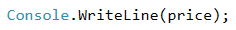# Problem: Transportation Price

A student has to travel n kilometers. He can choose between three types of transportation:

• Taxi. Starting fee: 0.70 EUR. Day rate: 0.79 EUR/km. Night rate: 0.90 EUR/km.
• Bus. Day / Night rate: 0.09 EUR/km. Can be used for distances of minimum 20 km.
• Train. Day / Night rate: 0.06 EUR/km. Can be used for distances of minimum 100 km.

Write a program that reads the number of kilometers n and period of the day (day or night) and calculates the price for the cheapest transport.

## Input Data

Two lines are read from the console:

• The first line contains a number n – number of kilometers – an integer in the range of [1 … 5000].
• The second line contains the word “day” or “night” – traveling during the day or during the night.

## Output Data

Print on the console the lowest price for the given number of kilometers.

## Sample Input and Output

Input Output Input Output
5
day
4.65 7
night
7
Input Output Input Output
25
day
2.25 180
night
10.8

## Hints and Guidelines

We will read the input data and depending on the distance, we will choose the cheapest transport. To do that, we will write a few conditional statements.

### Processing the Input Data

In the task, we are given information about the input and output data. Therefore, in the first two lines from the solution, we will declare and initialize the two variables that are going to store the values of the input data. The first line contains an integer and that is why the declared variable will be of int type. The second line contains a word, therefore, the variable will be of string type.Before starting with the conditional statements, we need to declare a variable that stores the value of the transport price.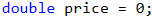### Calculating Taxi Rate

After having declared and initialized the input data and the variable that stores the value of the price, we have to decide which conditions of the task have to be checked first.

The task specifies that the rates of two of the vehicles do not depend on whether it is day or night, but the rate of one of the transports (taxi) depends. This is why the first condition will be whether it is day or night, so that it is clear which rate the taxi will be using. To do that, we declare one more variable that stores the value of the taxi rate.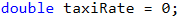In order to calculate the taxi rate, we will use conditional statement of type if-else and through it, the variable for the price of the taxi will store its value.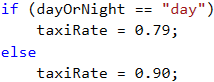### Calculating Transportation Price

After having done that, now we can start calculating the transport price itself. The constraints in the task refer to the distance that the student wants to travel. This is why, we will use an if-else statement that will help us find the price of the transport, depending on the given kilometers.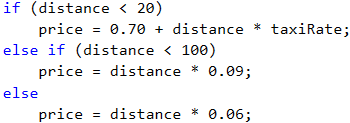First, we check whether the kilometers are less than 20, as the task specifies that the student can only use a taxi for less than 20 kilometers. If the condition is true (returns true), the variable that is created to store the value of the transport (price), will store the corresponding value. This value equals the starting fee that we will sum with its rate, multiplied by the distance that the student has to travel.

If the condition of the variable is not true (returns false), the next step of our program is to check whether the kilometers are less than 100. We do that because the task specifies that in this range, a bus can be used as well. The price per kilometer of a bus is cheaper than a taxi one. Therefore, if the result of the condition is true, we store a value, equal to the result of the multiplication of the rate of the bus by the distance to the variable for the transportation price in the else if statement body.

If this condition does not return true as a result, we have to store a value, equal to the result of the multiplication of the distance by the train rate to the price variable in the else body. This is done because the train is the cheapest transport for the given distance.

### Printing the Output Data

After we have checked the distance conditions and we have calculated the price of the cheapest transport, we have to print it. The task does not specify how to format the result, therefore, we just print the variable.